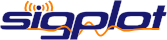fps
freq1
freq2
0 Hz 0 Hz

## Streaming/Real-time Plotting

For Layer1D, you can stream data to the plot by using the reload method.

```				```
plot_layer = plot.overlay_array(null, {
xdelta : xdelta,
xunits : 3,
yunits : 26,
size : fftsize / 2
});

// When new data comes in.
var data = [...];
```
```

Currently the data must be a Javascript array. The plot will automatically be scaled to the new data based on the 'autox' and 'autoy' options. However, when plotting a stream of data you may want to have the scaling adjust more smoothly. This can be done with the 'autol' option which specifies how many frames are averaged together when rescaling.

Often when using a plot to display streaming data you will also adjust the appearance (i.e. autohide panbars, etc.).

```				```
plot = new sigplot.Plot(document.getElementById('plot'), {all: true, expand: true, autol: 100, autohide_panbars: true});
```
```

For Layer2D, use the overlay_pipe() method and push()

```				```
raster_layer = raster.overlay_pipe({
type : 2000,
subsize : fftsize / 2,
xdelta : xdelta,
xunits : 3
});

// When new data comes in.
var data = [...];
plot.push(data_layer, data);
```
```